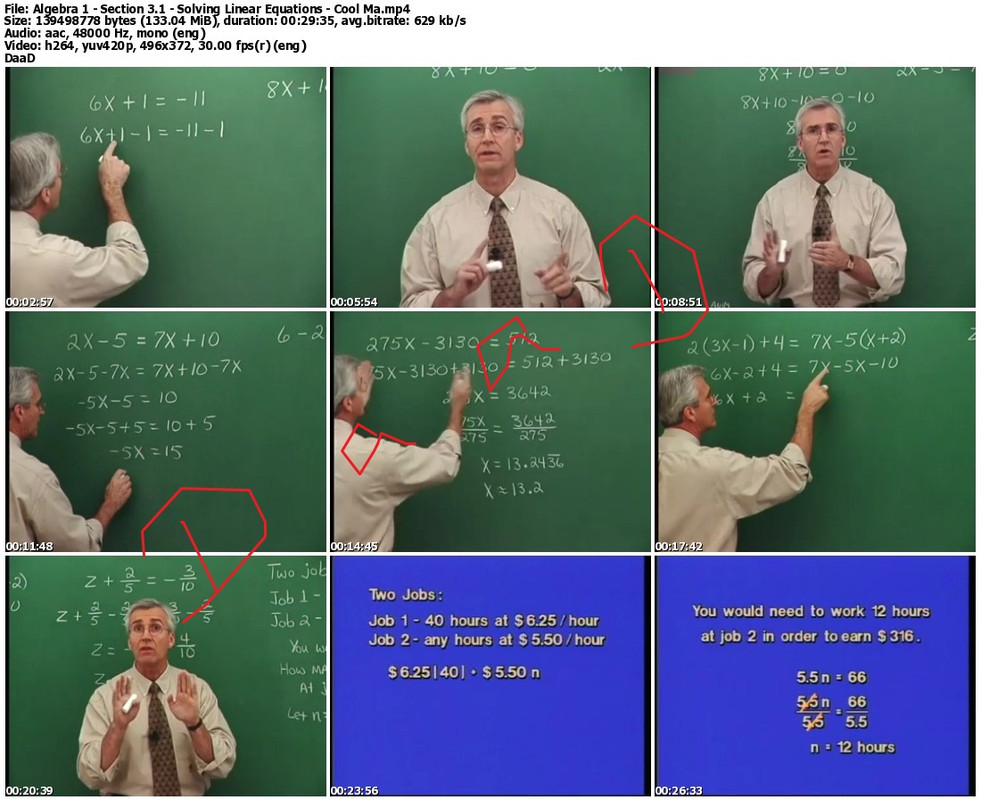## Cool Math Guy – Algebra [ I and II – Complete ]

Cool Math Guy – Algebra ICool Math Guy – Algebra I
DVDRips | MP4/AVC, ~499 kb/s | 496×372 | Duration: 21:00:09 | English: AAC, 117 kb/s (1 ch) | + PDF Guide
Size: 6.14 GB | Genre: Math

Core topics include solving linear equations and inequalities, graphing equations and inequalities with some use of the graphing calculator, exponents, polynomials, factoring, rational expressions and equations, systems of linear equations and inequalities, radical expressions and equations, and solving quadratic equations. Algebra I follows Prealgebra in the sequence of math courses and is often used as a developmental course at the college level under the name Elementary Algebra.

Chapter 1 – THE REAL NUMBER SYSTEM
1.1 Real Numbers: Order and Absolute Value
1.3 Multiplying and Dividing Integers
1.4 Operations with Rational Numbers
1.5 Exponents, Order of Operations, and Properties of Real Numbers

Chapter 2 – FUNDAMENTALS OF ALGEBRA
2.1 Writing and Evaluating Algebraic Expressions
2.2 Simplifying Algebraic Expressions
2.3 Algebra and Problem Solving
2.4 Introduction to Equations

Chapter 3 – LINEAR EQUATIONS AND PROBLEM SOLVING
3.1 Solving Linear Equations
3.2 Equations that Reduce to Linear Form
3.3 Problem Solving with Percents
3.4 Ratios and Proportions
3.5 Geometric and Scientific Applications
3.6 Linear Inequalities

Chapter 4 – EQUATIONS AND INEQUALITIES IN TWO VARIABLES
4.1 Ordered Pairs and Graphs
4.2 Graphs of Equations in Two Variables
4.3 Slope and Graphs of Linear Equations
4.4 Equations of Lines
4.5 Graphs of Linear Inequalities
Chapter 5 – EXPONENTS AND POLYNOMIALS

5.1 Negative Exponents and Scientific Notation
5.3 Multiplying Polynomials: Special Products
5.4 Dividing Polynomials

Chapter 6 – FACTORING AND SOLVING EQUATIONS
6.1 Factoring Polynomials with Common Factors
6.2 Factoring Trinomials
6.4 Factoring Polynomials with Special Forms
6.5 Solving Quadratic Equations by Factoring

Chapter 7 – RATIONAL EXPRESSIONS AND EQUATIONS
7.1 Simplifying Rational Expressions
7.2 Multiplying and Dividing Rational Expressions
7.3 Adding and Subtracting Rational Expressions
7.4 Complex Fractions
7.5 Rational Equations and Applications

Chapter 8 – SYSTEMS OF LINEAR EQUATIONS AND INEQUALITIES
8.1 Solving Systems of Equations by Graphing
8.2 Solving Systems of Equations by Substitution
8.3 Solving Systems of Equations by Elimination
8.4 Applications of Systems of Linear Equations
8.5 Systems of Linear Inequalities

Chapter 9 – RADICAL EXPRESSIONS AND EQUATIONS

Chapter 10 – QUADRATIC EQUATIONS AND FUNCTIONS
10.1 Solution by the Square Root Property
10.2 Solution by Completing the Square
10.3 Solution by the Quadratic Formula
10.6 Complex Numbers
10.7 Relations, Functions, and Graphs`https://rapidgator.net/file/7b266b23fdbd6d5d9506c6de4c417843/dfh78C00lMthGuyAlgbra01.part1.rar.htmlhttps://rapidgator.net/file/b2d04eb22282136a1bd73bed02a3e9d1/dfh78C00lMthGuyAlgbra01.part2.rar.htmlhttps://rapidgator.net/file/d390a7c63f5b1c780ca4ccf17e3cf3e1/dfh78C00lMthGuyAlgbra01.part3.rar.htmlhttps://rapidgator.net/file/4946b60777045733fa096b27fa329f55/dfh78C00lMthGuyAlgbra01.part4.rar.html`

Cool Math Guy – Algebra IITopics in Algebra II primarily extend concepts learned in Algebra I and also include functions, complex numbers, exponential and logarithmic functions, use of the graphing calculator, matrices, conics, sequences and series. Algebra II follows either Algebra I or Geometry in the sequence of math courses and is often used as a developmental course at the college level under the name Intermediate Algebra.

Chapter 1 – FUNDAMENTALS OF ALGEBRA
1.1 The Real Number System
1.2 Operations with Real Numbers
1.3 Properties of Real Numbers
1.4 Algebraic Expressions
1.5 Constructing Algebraic Expressions

Chapter 2 – LINEAR EQUATIONS AND INEQUALITIES
2.1 Linear Equations
2.2 Linear Equations and Problem Solving
2.4 Linear Inequalities
2.5 Absolute Value Equations and Inequalities

Chapter 3 – GRAPHS AND FUNCTIONS
3.1 The Rectangular Coordinate System
3.2 Graphs of Equations
3.3 Slope and Graphs of Linear Equations
3.4 Equations of Lines
3.5 Graphs of Linear Inequalities
3.6 Relations and Functions
3.7 Graphs of Functions

Chapter 4 – SYSTEMS OF EQUATIONS AND INEQUALITIES
4.1 Systems of Equations
4.2 Linear Systems in Two Variables
4.3 Linear Systems in Three Variables
4.4 Matrices and Linear Systems
4.5 Determinants and Linear Systems
4.6 Systems of Linear Inequalities

Chapter 5 – POLYNOMIALS AND FACTORING
5.1 Integer Exponents and Scientific Notation
5.3 Multiplying Polynomials
5.4 Factoring by Grouping and Special Forms
5.5 Factoring Trinomials
5.6 Solving Polynomial Equations by Factoring

Chapter 6 – RATIONAL EXPRESSIONS, EQUATIONS, AND FUNCTIONS
6.1 Rational Expressions and Functions
6.2 Multiplying and Dividing Rational Expressions
6.3 Adding and Subtracting Rational Expressions
6.4 Complex Fractions
6.5 Dividing Polynomials and Synthetic Division
6.6 Solving Rational Equations
6.7 Applications and Variation

Chapter 7 – RADICALS AND COMPLEX NUMBERS
7.4 Multiplying and Dividing Radical Expressions
7.6 Complex Numbers

Chapter 8 – QUADRATIC EQUATIONS, FUNCTIONS, AND INEQUALITIES
8.1 Solving Quadratic Equations: Factoring and Special Forms
8.2 Completing the Square

Chapter 9 – EXPONENTIAL AND LOGARITHMIC FUNCTIONS
9.1 Exponential Functions
9.2 Composite and Inverse Functions
9.3 Logarithmic Functions
9.4 Properties of Logarithms
9.5 Solving Exponential and Logarithmic Equations
9.6 Applications

Chapter 10 – CONICS
10.1 Circles and Parabolas
10.2 Ellipses
10.3 Hyperbolas
10.4 Solving Nonlinear Systems of Equations

Chapter 11 – SEQUENCES, SERIES, AND THE BINOMIAL THEOREM
11.1 Sequences and Series
11.2 Arithmetic Sequences
11.3 Geometric Sequences and Series
11.4 The Binomial Theorem`https://rapidgator.net/file/f37403c9fbbe0bf235e7ddc3fc9636ff/fgj67C00lMthGuyAlgbra2.part1.rar.htmlhttps://rapidgator.net/file/d68ff0d9c538e38a1bd8af2ad6b77825/fgj67C00lMthGuyAlgbra2.part2.rar.htmlhttps://rapidgator.net/file/8ee45c6a46fa7b175bfa332bbd5f7221/fgj67C00lMthGuyAlgbra2.part3.rar.htmlhttps://rapidgator.net/file/879bab09aa585942defd86c4063e7ad1/fgj67C00lMthGuyAlgbra2.part4.rar.htmlhttps://rapidgator.net/file/b34836abc15fe525414ede391911047b/fgj67C00lMthGuyAlgbra2.part5.rar.html`